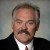# Can you explain "degrees of freedom" in the context of a Six Sigma tool, such as a DOE?

ByDr. Mikel Harry

Let us suppose we are interested in testing the influence of a certain independent variable (say, A) as it relates to a given dependent variable (say, Y).  We will suppose the experimental factor (variable A) is to be tested at two competing settings (test levels).  In this scenario, the two levels will be called “high” and low,” respectively.

For purposes of this discussion, we will state (for the record) that the aforementioned experiment was properly designed and executed.  Given this, we will now consider the one-way analysis-of-variance procedure (also called One-Way ANOVA).  The informed reader will thoroughly understand that One-Way ANOVA is intended to provide a means by which the total observed sums-of-squares can be partitioned into its respective contributing parts, also called “components of variation.”

In abbreviated form, we must first establish or otherwise account for the total variations present during the course of experimentation.  This is called the “total sums-of-squares,” and most often provided in the form SST.  Second, we concede that portion of SST that is attributable to the experimental variable (i.e., factor A), generally referred to as the “between-group sums-of-squares” and appropriately designated as SSB.  Third, we acknowledge the residual error, commonly referenced as the “within-group sums-of-squares,” or simply SSW.  Thus, we understand that SST = SSB + SSW (so long as the covariance is zero).  This is to say that the total experimental error can be explained by two fundamental components – first, the aggregate of those variations observed “between test conditions” and second, the aggregate of those variations occurring “within test conditions.”

Handpicked Content:   In Minitab on the Poisson plot it plots expected defects versus observed defects. Where do the expected defects come from?

From our example’s point of view, we naturally express the total degrees of freedom as df.T = ng –1, where n is the common number of replicates associated with each test condition and g is the number of such test conditions being considered.  Given the composite set of variations across the entire experiment (SST), we separate those that are observed when variable A is at its high level from those when A is at its low level.  Of course, these two independent sets of variations are statistically pooled into one, thereby forming SSW.

Consequently, the within-group degrees-of-freedom associated with this particular component of variation is given as df.W = g(n –1).  Of course, we know by theoretical constructs that df.T = df.B + df.W.  By way of some simple algebra, it can be readily demonstrated that df.B = df.T – df.W.  Hence, df.B = g –1.  For our example, we determine the between-group degrees-of-freedom to be df.B = g –1 = 2 –1 = 1. Thus, we conclude there exists one degree-of-freedom with which to estimate the influence of variable A on Y.  Hence, we have the application of this concept to design-of-experiments (DOE).

For a more detailed understanding relating to degrees-of-freedom, as given in the context of a statistically designed experiment, you should reference one of the well-known textbooks in this field of endeavor (such as that offered by Montgomery or Box).  If you are seeking a more theoretical understanding, then perhaps you should consult the “Handbook of Applied Mathematics” by Pearson.  Of course, there is much available on the Internet, although you may have to dig though a lot of junk to find the jewels.